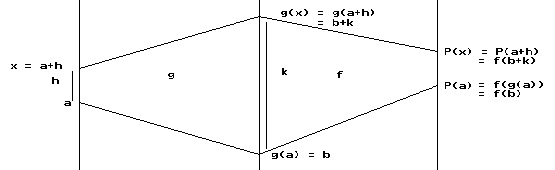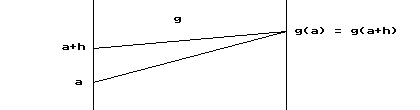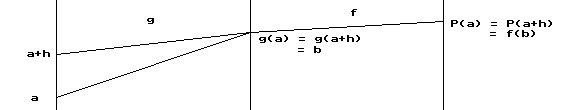Chain Rule Proof Theorem (Chain Rule): If g is differentiable at a and f is differentiable at b=f(a), and h(x) = f(g(x)) for an interval I with a in I
then h is differentiable at a and h'(a) = f'(g(a)) cdot g'(a).

PROOF of Chain Rule:
Part I: Assume there is some interval ,I containing a where for all x in I , g(x) ne g(a).Let b = g(a)and k = g(a+h) - g(a) = g(x) - g(a) for h ne 0.
Note g(x) = g(a+h) = g(a) + k = b + k. See Figure 1 .

From the assumption that g is differentiable at a, we have that g is also continuous at a . Thus we can conclude that as h to 0, k to 0.

We’ll follow the usual steps in finding the derivative of P at a:
Step I: P(a+h) = f(g(a+h))
\underline{- P(a)\ \  \ \  = f(g(a))}
Step II: P(a+h) - P(a) = f(g(a+h)) - f(g(a)) = f(b + k) - f(b).
Now we assumed that k ne 0 . [Note: This is a major assumption for some functions.]
So
P(a+h) - P(a) = {f(b + k) - f(b)}/k . k
Therefore
P'(a) = lim_{h to 0} { P(a+h) - P(a)}/h
= lim _{h to 0,  k to 0} {f(b + k) - f(b)}/k  cdot  k/h
= lim _{h to 0,  k to 0} {f(b + k) - f(b)}/k  cdot {g(a+h)-g(a) }/h
= f '(b)  cdot g'(a)
= f '(g(a)) cdot g'(a).

Part II:  Recall that we had k = g(a+h)-g(a) for h ne 0.
Suppose that  k = 0 for values of h arbitrarily close to 0.
Since we assume that g is differentiable we know thatlim_{h to 0} {g(a+h)-g(a)}/ h must exist. Our assumption that k = 0 for h arbitrarily close to 0 means that
there is a sequence of  values of  h, {h_n} with h_n to 0 and  g(a+h_n) -g(a) = 0 for all n.
Thus lim_{n  to oo} {g(a+h_n)-g(a)}/ {h_n } = lim_{n  to oo} 0/ {h_n }= 0 . [See Figure 2 ]
Thus  g'(a) = lim_{ h to 0} {g(a+h)-g(a)} / h = 0. [0 is the only possible limit.]

To complete the argument we need only show that P'(a)= 0.
But for precisely the same h values that had k = g(a+h)-g(a) = 0, we have g(a+h) = g(a). Thus for these values of h
P(a+h) - P(a) = f(g(a+h)) - f(g(a)) = f(b + k) - f(b) = f(b) - f(b) = 0.and hence {P(a+h) - P(a)}/h = 0. [See Figure 3]

Now for any h where k ne 0, see Figure 1, the argument of  part I is still valid to show that {P(a+h) - P(a)}/h to 0 as h  to 0.

[This is primarily because g'(a)=0.]

In summary then , as h approaches 0 either {P(a+h)-P(a)}/h is
close to or actually is 0.
Thus P'(a) =lim_{h to 0} {P(a+h) - P(a) }/h= 0. EOP.